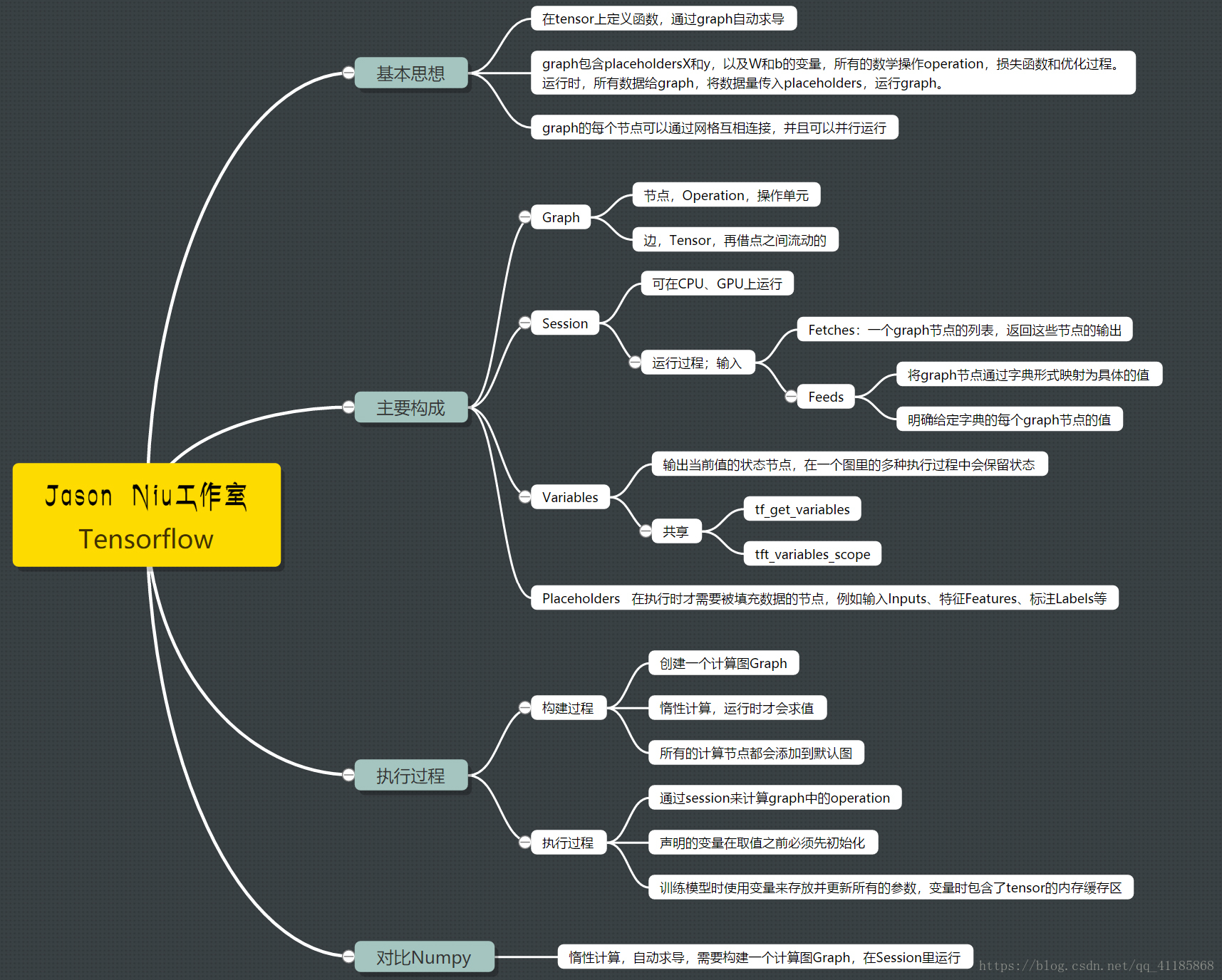TensorFlow 学习指南 二、线性模型

+关注继续查看

广播

import tensorflow as tf

a = tf.constant(3, name='a')

with tf.Session() as session:
print(session.run(a))

a = tf.constant(3, name='a')
b = tf.constant(4, name='b')

with tf.Session() as session:

a = tf.constant([1, 2, 3], name='a')
b = tf.constant([4, 5, 6], name='b')

with tf.Session() as session:

a = tf.constant([1, 2, 3], name='a')
b = tf.constant(4, name='b')

with tf.Session() as session:

a = tf.constant([[1, 2, 3], [4, 5, 6]], name='a')
b = tf.constant([[1, 2, 3], [4, 5, 6]], name='b')

with tf.Session() as session:

a = tf.constant([[1, 2, 3], [4, 5, 6]], name='a')
b = tf.constant(100, name='b')

with tf.Session() as session:

a = tf.constant([[1, 2, 3], [4, 5, 6]], name='a')
b = tf.constant([100, 101, 102], name='b')

with tf.Session() as session:

a = tf.constant([[1, 2, 3], [4, 5, 6]], name='a')
b = tf.constant([100, 101,], name='b')

with tf.Session() as session:

a = tf.constant([[1, 2, 3], [4, 5, 6]], name='a')
b = tf.constant([, ], name='b')

with tf.Session() as session:

a.shape
TensorShape([Dimension(2), Dimension(3)])
b.shape
TensorShape([Dimension(2), Dimension(1)])

随机性

TensorFlow 有许多用于生成随机数的内置方法。这包括我们熟悉的分布，如“均匀”，以及你可能听说过的其他分布，如“正态”分布。均匀分布就像你掷骰子时得到的东西那样 - 有一组值，它们都是等可能的。正态分布是统计课程中教授的标准，其中数据具有更可能的平均值，以及围绕它的“钟形”曲线。我们将看到的，其他的也包括在内。

import tensorflow as tf

def run_variable(variable):
tf.initialize_all_variables()
with tf.Session() as sess:
return sess.run(variable)

my_distribution = tf.random_uniform((6, 4), seed=42)
uniform = run_variable(my_distribution)

from matplotlib import pyplot as plt

plt.hist(uniform.flatten())
plt.show()large_normal = tf.random_uniform((600, 400), seed=42)
large_uniform = run_variable(large_normal)

plt.hist(large_uniform.flatten())
plt.show()distribution = tf.random_normal((600, 4), seed=42)
normal = run_variable(distribution)
plt.hist(normal.flatten())
plt.show()distribution = tf.random_normal((10000,), seed=42, mean=170, stddev=15)
normal = run_variable(distribution)
plt.hist(normal.flatten())
plt.show()import numpy as np
bins = tf.histogram_fixed_width(normal, (normal.min(), normal.max()), nbins=20)
histogram_bins = run_variable(bins)
x_values = np.linspace(normal.min(), normal.max(), len(histogram_bins))
plt.bar(x_values, histogram_bins,)

plt.bar调用中，我们再次手动生成bin值，然后使用条形图将这些值绘制为x值，并使用histogram_bins作为高度。bar_width = (normal.max() - normal.min()) / len(histogram_bins)
plt.bar(x_values, histogram_bins, width=bar_width)• 使用均匀分布建模单次掷骰子。 绘制结果来确保其符合你的期望
• 使用单个图中的纯 TensorFlow 调用替换本课程的最后一个代码块。 换句话说，使用 TensorFlow 概念来替换.min(),.max()len调用。 只有绘图在没有 TensorFlow 的情况下进行！

线性方程import tensorflow as tf

# 点 1
x1 = tf.constant(2, dtype=tf.float32)
y1 = tf.constant(9, dtype=tf.float32)
point1 = tf.stack([x1, y1])

# 点 2
x2 = tf.constant(-1, dtype=tf.float32)
y2 = tf.constant(3, dtype=tf.float32)
point2 = tf.stack([x2, y2])

# 将点组合为数组
X = tf.transpose(tf.stack([point1, point2]))B = tf.ones((1, 2), dtype=tf.float32)

parameters = tf.matmul(B, tf.matrix_inverse(X))

with tf.Session() as session:
A = session.run(parameters)

b = 1 / A
a = -b * A
print("Equation: y = {a}x + {b}".format(a=a, b=b))import tensorflow as tf

points = tf.constant([[2, 1],
[0, 5],
[-1, 2]], dtype=tf.float64)

A = tf.constant([
[2, 1, 1],
[0, 5, 1],
[-1, 2, 1]
], dtype='float64')

B = -tf.constant([, , ])

X = tf.matrix_solve(A, B)

with tf.Session() as session:
result = session.run(X)
D, E, F = result.flatten()

print("Equation: x**2 + y**2 + {D}x + {E}y + {F} = 0".format(**locals()))

1）求解包含以下三点的圆：P(2,1), Q(0,5), R(-1,2)

2）下面给出椭圆的一般形式。 解决以下几点（解决这个方程需要五点）：3D 中的 TensorFlow

TensorFlow 不仅仅是一个深度学习库 - 它是一个但数值操作库，因此它可以执行许多其他库可以执行的任务。 在本课中，我们将介绍如何使用 TensorFlow 对 3D 对象执行操作。

3D 对象可以被建模为三维空间中的一系列三角形，我们通常将其称为(x, y, z)。 这些名称不是必需的，但通常使用。 从这些 3D 点中的三个创建三角形。 点本身可以表示为大小为(3,)的向量。 这些数组是一个大小为(n, 3),的矩阵，其中n是我们拥有的点数。 让我们深入去看一个基本的立方体。 我们稍后将需要此功能，所以让我们创建一个绘制基本形状的函数：

from mpl_toolkits.mplot3d import Axes3D
import numpy as np
from matplotlib import cm
import matplotlib.pyplot as plt
from scipy.spatial import Delaunay
​
def plot_basic_object(points):
"""绘制一个基本对象，假设它是凸的而不是太复杂"""

tri = Delaunay(points).convex_hull
fig = plt.figure(figsize=(8, 8))
S = ax.plot_trisurf(points[:,0], points[:,1], points[:,2],
triangles=tri,
ax.set_xlim3d(-5, 5)
ax.set_ylim3d(-5, 5)
ax.set_zlim3d(-5, 5)

plt.show()

%matplotlib notebook

import numpy as np
def create_cube(bottom_lower=(0, 0, 0), side_length=5):
"""从给定的左下角点（最小的 x，y，z 值）开始创建一个立方体"""
bottom_lower = np.array(bottom_lower)
points = np.vstack([
bottom_lower,
bottom_lower + [0, side_length, 0],
bottom_lower + [side_length, side_length, 0],
bottom_lower + [side_length, 0, 0],
bottom_lower + [0, 0, side_length],
bottom_lower + [0, side_length, side_length],
bottom_lower + [side_length, side_length, side_length],
bottom_lower + [side_length, 0, side_length],
bottom_lower,
])
return points

cube_1 = create_cube(side_length=2)
​
​
plot_basic_object(cube_1)平移

import tensorflow as tf

def translate(points, amount):

points = tf.constant(cube_1, dtype=tf.float32)# 更新此处的值来移动多维数据集。
translation_amount = tf.constant([3, -3, 0], dtype=tf.float32)

translate_op = translate(points, translation_amount)with tf.Session() as session:
translated_cube = session.run(translate_op)

plot_basic_object(translated_cube)沿x轴旋转

[[1, 0, 0],
[0, cos \theta, sin \theta],
[0, -sin \theta, cos \theta]]

沿y轴旋转

[[cos \theta, 0, -sin \theta],
[0, 1, 0],
[sin \theta, 0, cos \theta]]

沿z轴旋转

[[cos \theta, sin \theta, 0],
[-sin \theta, cos \theta, 0],
[0, 0, 1]]
def rotate_around_z(points, theta):
theta = float(theta)
rotation_matrix = tf.stack([[tf.cos(theta), tf.sin(theta), 0],
[-tf.sin(theta), tf.cos(theta), 0],
[0, 0, 1]])
return tf.matmul(tf.to_float(points), tf.to_float(rotation_matrix))

with tf.Session() as session:
result = session.run(rotate_around_z(cube_1, 75))

plot_basic_object(result)• 创建不同的对象，例如四棱锥或者六棱柱。 如果你不确定如何开始，请先从棱柱开始，然后先在2D中创建它。
• 围绕x轴和y轴旋转对象。
• 你可以将旋转组合到单个变换矩阵中。 为此，只需计算旋转的点积。
• 对于问题 3，顺序是否重要？
• 剪切矩阵是具有非对角线值的单位矩阵。 一个例子如下。 创建剪切矩阵并测试不同的值。
[[1, 0.5, 0],
[0, 1, 0],
[0, 0, 1]]

线性模型的分类

from sklearn.datasets import make_blobs

import numpy as np

from sklearn.preprocessing import OneHotEncoder

X_values, y_flat = make_blobs(n_features=2, n_samples=800, centers=3, random_state=500)
y = OneHotEncoder().fit_transform(y_flat.reshape(-1, 1)).todense()
y = np.array(y)

%matplotlib inline

from matplotlib import pyplot as plt

# 可选的行：将默认数字大小设置得稍大。
plt.rcParams['figure.figsize'] = (24, 10)

plt.scatter(X_values[:,0], X_values[:,1], c=y_flat, alpha=0.4, s=150)from sklearn.model_selection import train_test_split

X_train, X_test, y_train, y_test, y_train_flat, y_test_flat = train_test_split(X_values, y, y_flat)

X_test += np.random.randn(*X_test.shape) * 1.5

#plt.scatter(X_train[:,0], X_train[:,1], c=y_train_flat, alpha=0.3, s=150)
plt.plot(X_test[:,0], X_test[:,1], 'rx', markersize=20)创建模型

​Y = XW + b

X中的点是固定的 - 这些是训练或测试数据，称为观测数据。 Wb的值是我们模型中的参数，我们可以控制这些值。 为这些值选择好的值，可以为我们提供良好的决策线。

import tensorflow as tf

n_features = X_values.shape
n_classes = len(set(y_flat))

weights_shape = (n_features, n_classes)

W = tf.Variable(dtype=tf.float32, initial_value=tf.random_normal(weights_shape))  # Weights of the model

X = tf.placeholder(dtype=tf.float32)

Y_true = tf.placeholder(dtype=tf.float32)

bias_shape = (1, n_classes)
b = tf.Variable(dtype=tf.float32, initial_value=tf.random_normal(bias_shape))

Y_pred = tf.matmul(X, W)  + b

Y_pred中的实际值由“似然”组成，模型将为给定点选择每个类的似然，生成(n_rows， n_classes)大小的矩阵。它们不是真正的似然，但我们可以通过找到最大值，来找出我们的模型认为的最有可能的类。

loss_function = tf.losses.softmax_cross_entropy(Y_true, Y_pred)

with tf.Session() as sess:
sess.run(tf.global_variables_initializer())
for i in range(5000):
result = sess.run(learner, {X: X_train, Y_true: y_train})
if i % 100 == 0:
print("Iteration {}:\tLoss={:.6f}".format(i, sess.run(loss_function, {X: X_test, Y_true: y_test})))
y_pred = sess.run(Y_pred, {X: X_test})
W_final, b_final = sess.run([W, b])

predicted_y_values = np.argmax(y_pred, axis=1)
predicted_y_values

h = 1
x_min, x_max = X_values[:, 0].min() - 2 * h, X_values[:, 0].max() + 2 * h
y_min, y_max = X_values[:, 1].min() - 2 * h, X_values[:, 1].max() + 2 * h
x_0, x_1 = np.meshgrid(np.arange(x_min, x_max, h),
np.arange(y_min, y_max, h))
decision_points = np.c_[x_0.ravel(), x_1.ravel()]

# 我们在 NumPy 中重建我们的模型
Z = np.argmax(decision_points @ W_final[[0,1]] + b_final, axis=1)

# 创建 x_0 和 x_1 值的等高线图
Z = Z.reshape(xx.shape)
plt.contourf(x_0, x_1, Z, alpha=0.1)

plt.scatter(X_train[:,0], X_train[:,1], c=y_train_flat, alpha=0.3)
plt.scatter(X_test[:,0], X_test[:,1], c=predicted_y_values, marker='x', s=200)

plt.xlim(x_0.min(), x_0.max())
plt.ylim(x_1.min(), x_1.max())• 绘制迭代和损失之间的关系。 出现什么样的形状，你认为它将如何继续？
• 使用 TensorBoard，将图写入文件，并查看 TensorBoard 中变量的值。 更多信息请参阅其余教程。
• 通过在传递到线性模型之前对X执行一些变换来创建非线性模型。 这可以通过多种方式完成，你的模型的准确性将根据你的选择而改变。
• 使用以下代码加载 64 维（称为数字）的数据集，并将其传递给分类器。 你得到了什么预测准确度？
X = digits.data
y = digits.target2113 010099 010887 0DL框架之Tensorflow：深度学习框架Tensorflow的简介、安装、使用方法之详细攻略（二）
DL框架之Tensorflow：深度学习框架Tensorflow的简介、安装、使用方法之详细攻略
24 013896 0【干货】史上最全的Tensorflow学习资源汇总

2180 0TensorFlow学习笔记之五——源码分析之最近算法
import numpy as np import tensorflow as tf # Import MINST data import input_data mnist = input_data.read_data_sets("/tmp/data/", one_hot=True) #这里主要是导入数据，数据通过input_data.py已经下载到/tmp/data/目录之下了，这里下载数据的时候，需要提前用浏览器尝试是否可以打开 #http://yann.lecun.com/exdb/mnist/，如果打不开，下载数据阶段会报错。
1116 0《白话深度学习与TensorFlow》——3.3　其他特点

1927 0【翻译】Sklearn与TensorFlow机器学习实用指南 —— 第16章 强化学习（下）
1616 0
apachecn_飞龙
Github：@wizardforcel 简书：@ApacheCN_飞龙 微博：@龙雀 CSDN：@wizardforcel ApacheCN 官网：apachecn.org 机器学习交流群：629470233
719

0

《2021云上架构与运维峰会演讲合集》

《零基础CSS入门教程》

《零基础HTML入门教程》